Hello and welcome to this new project blog where you'll learn to create a Digital Spirit Level.
A traditional spirit level is a simple device used for the detection of the level of the surface, whether the surface is horizontal or vertical. There are many different types of spirit level. which are used in various fields but their main purpose is common between all of them. The traditional spirit levels have some issues that are faced by the users like the spirit level is not very accurate and can’t measure the different surface angles and they are not handy on vibrating surfaces as well. To resolve these problems, we are making a digital spirit level that can measure surface angle very accurately and can do many more things which have been discussed in detail below.

Components Required

Target Of This Project

The target of this project is to make a reliable system to measure the angle of surface in different circumstances which can be directly used in industrial applications and areas and not being expensive at the same time.
Digital Spirit Level has several features, Such as:-

● It can measure the surface angle in two axes.
● It can show the surface angle of each axis at different as well as at the same time.
● Accuracy is about 土 0.1 degree.
● Delay time to show a correct surface angle is less than 1 second.
● Offset feature is available for both axes.
● It also can show the temperature of the surrounding area.
● A charging and direct USB powering system are available.
● A battery level indicator on the display screen is available.
● Negligible error due to temperature change.
● Very few errors in measurement insignificant vibrations.
● A buzzer is installed to produce a beep sound whenever the push button is pressed.
● The 3D printed casing is given to make it robust and easy to use.

Technology Used

Process and control system:-
For the data processing from the sensor, input command from push buttons, display of data on the screen, and controlling the system, all is done by the single Arduino Nano development board. Which is the very famous 8-bit AVR microcontroller based on RISC architecture.
This microcontroller is chosen because no. of I/O pins are more than sufficient, the required communication protocol is available like I2C and UART, good processing speed which is enough for our spirit level, and most important they have a good support environment like they provide Arduino IDE for high-level programming and forum to resolve doubts.

The sensor part of the system:-
For measuring the angle of the surface, we have used a sensor that can sense any type of acceleration or rotation. So, we have chosen a very low-cost MPU6050 based on Micro-Electro-Mechanical Systems (MEMS) technology and has six degrees of freedom.
That means it consists of a 3-axis accelerometer and a 3-axis gyroscope. MPU6050 uses inter-integrated circuit (I2C) communication protocol to communicate with microcontrollers.

Before moving forward we are going to understand the Accelerometer and Gyroscope in detail and understand how they work.

• MPU6050 Accelerometer:- The MPU6050 consists of a 3-axis Accelerometer with Micro Electro Mechanical (MEM) technology. The MPU-6050 architecture reduces the chances of an accelerometer going into a state of thermal drift. Acceleration along the axes deflects the movable mass. This displacement of moving plate (mass) unbalances the differential capacitor which results in sensor output. 16-bit ADC is used to get digitized output. The full-scale range of acceleration is +/- 2g, +/- 4g, +/- 8g, +/- 16g, where g is the gravity force unit. When an accelerometer is placed on a plane surface it will measure 0g on X and Y axes, and +1g on the z-axis.
• MPU6050 Gyroscope:- The MPU-6050 consists of three independent vibratory MEMS rate gyroscopes, which detect rotation about the X, Y, and Z axes. When the gyros are rotated about any of the sense axes, the Coriolis Effect causes a vibration that is detected by a MEM inside MPU6050. The resulting signal is amplified, demodulated, and filtered to produce a voltage that is proportional to the angular rate. This voltage is digitized using 16-bit ADC to sample each axis. The full-scale range of the gyro sensors may be digitally programmed to ±250, ±500, ±1000, or ±2000 degrees per second (DPS). It measures the angular velocity along each axis in degrees per the second unit.

Display system:- For displaying the surface angle on the screen we are using the SSD1306 0.96 inches Organic Light Emitting Diode(OLED) which is a light-emitting diode (LED) in which the emissive electroluminescent layer is a film of organic compound that emits light in response to an electric current. It is chosen due to low power consumption and wide view angle. It’s available in I2C or 3-wire SPI protocol for communicating with microcontrollers.
For our digital spirit level, we are using I2C protocol-based OLED to keep it more simple and handy with wire connections.

Workflow chart and Procedure

Workflow chart:-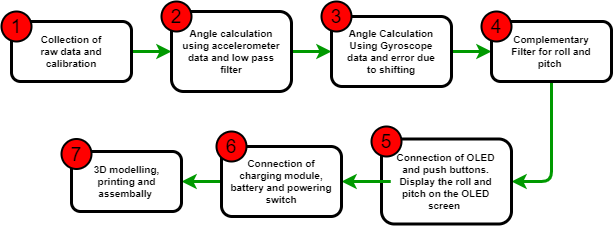Step 1:- Collection of raw data from MPU6050 and its calibration

Connection of the Arduino nano with MPU6050:-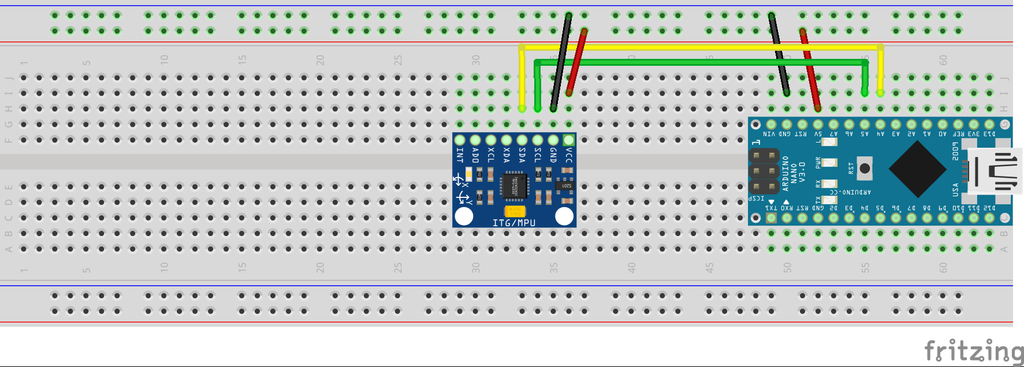```#include "I2Cdev.h"
#include "MPU6050.h" // MPU6050 Library
MPU6050 mpu; //Creating Object for MPU6050
int16_t gyroX, gyroY, gyroZ, accX, accY, accZ, mpuTemp; //Variable to collect the raw data from MPU6050 Sensor
float CgyroX, CgyroY, CgyroZ, CaccX, CaccY, CaccZ; //Calibrated DATA variables
float Offset_AccX,Offset_AccY,Offset_AccZ,Offset_GyroX,Offset_GyroY,Offset_GyroZ; //Verialble for offset value
void setup() {
// put your setup code here, to run once:
Serial.begin(9600);
OFFSET();
}
void loop() {
// put your main code here, to run repeatedly:
RAW_DATA();
CALEBRATED_DATA()
}
// To Calculate the Offset Error
void OFFSET()
{
for(int i = 0; i&lt;200; i++) // To calculate the offset value by average of 200 data
{
mpu.getMotion6(&amp;accX, &amp;accY, &amp;accZ, &amp;gyroX, &amp;gyroY, &amp;gyroZ);
Offset_AccX += accX;
Offset_AccY += accY;
Offset_AccZ += accZ;
Offset_GyroX += gyroX;
Offset_GyroY += gyroY;
Offset_GyroZ += gyroZ;
}
Offset_AccX = Offset_AccX/200;
Offset_AccY = Offset_AccY/200;
Offset_AccZ = Offset_AccZ/200;
Offset_GyroX = Offset_GyroX/200;
Offset_GyroY = Offset_GyroY/200;
Offset_GyroX = Offset_GyroZ/200;
mpu.setXAccelOffset(Offset_AccX);
mpu.setYAccelOffset(Offset_AccY);
mpu.setZAccelOffset(Offset_AccZ);
mpu.setXGyroOffset(Offset_GyroX);
mpu.setYGyroOffset(Offset_GyroY);
mpu.setZGyroOffset(Offset_GyroZ);
}
void RAW_DATA()
{
mpu.getMotion6(&amp;accX, &amp;accY, &amp;accZ, &amp;gyroX, &amp;gyroY, &amp;gyroZ);
}
void CALEBRATED_DATA()
{
//To Get the GYRO DATA in the range of +-250°/s
CgyroX = gyroX/131.0;
CgyroY = gyroY/131.0;
CgyroZ = gyroZ/131.0;
//To get the ACC. DATA in the range of +-2g
CaccX = accX/16384.0;
CaccY = accY/16384.0;
CaccZ = accZ/16384.0;
}
```

As we know, MPU6050 communicates through the I2C protocol on a unique I2C address named - 0x68. Due to this, we establish the connection through SCL(A5) and SDA(A4) pins, and for power, the VCC pin is connected to 5V and GND Pin to GND of the Arduino.

Here we have two methods for Collecting the Raw data:-
1. Manually addressing each register, where we can define individual registers with their unique address for stabilizing the proper I2C communication between Arduino and MPU6050.
2. Another method is to use the Library for MPU6050, where we have predefined functions to calculate the raw data and manipulate it as well. We are using the "MPU6050.h" Library for MPU6050 and "I2Cdev.h" Library for I2C Communication.

For Obtaining Raw data, Calibrated data, and Offset value, we have written the following Code Segment:-

As we know, In every sensor there exists a certain amount of offset, to get our readings accurately we need to calculate the offset Error and subtract from the later data.
Here, the inbuilt offset function of the "MPU6050.h" library Subtracts the offset error from the later data.
To Calculate the Offset Error, we are taking readings 200-300 times of the sensor at the stationary position to obtain the correct offset.
Now, we can print calibrated data of the 3-axis accelerometer and 3-axis gyroscope on the serial monitor of Arduino IDE.

Step-2:- Calculation of Angle using Accelerometer and Applying Low-pass Filter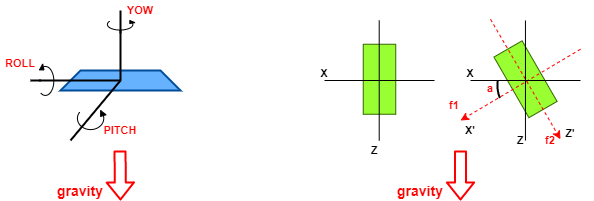As we know, we can find the angle only using the gravitational force components in two axes( Separated at 90 degrees) which act on the body. Therefore, we need a 3-axis accelerometer that can sense the gravitational force on its 3 different axes.
Before going to the calculation of the surface angle we should know some more basics. The direction of gravity never changes. So, if we change the angle by rotating the sensor about a parallel axis to the gravity( YOW angle) then we will not get any change in any 3-axes of the sensor. ROLL and PITCH angles can change because the gravitational force will split into two vector components that can sense by the different axis of the accelerometer.
If we see the green body in the above figure, initially the body is making a zero degree angle with the x-axis. While after rotation about the PITCH axis we can see the gravitational force is split into two components.

g = f1 + f2

And we can find the angle with a simple trigonometric ratio:-

a = arctan(f1/f2)

The code for calculating the angle from Raw accelerometer data:-

//Function to calculate the angle from the RAW Accelerometer Date
void ACC_ANGLE_CALCULATION()
{ //Angle Calculation --> Arc Tangent(X-gravity/Z-gravity)*(RADIAN TO DEGREE)
}

The angle is coming only from the MPU6050 accelerometer data, which can have some noise. Or we can say that the calculated angle can be affected by some vibration acceleration and disturbance. So, we are developing the code for a low pass filter. Which depend 96% on previous data and 4% present data to minimize the sudden changes in accelerometer data.

//Low pass filter to filter the noise coming from the disturbance
void LOW_PASS_FILTER()
{ // [NEW_FILTER_DATA = a*OLD_FILTER_DATA + b*ACTUAL DATA] and [a + b = 1]
FNXang = (0.96*FOXang + 0.04*Xang); //ACCELEROMETER_ROLL
FNYang = (0.96*FOYang + 0.04*Yang); //ACCELEROMETER_PITCH
}
//To update all previous data with new data
void UPDATE()
{
//Updating the old filter value with new filter value
FOXang = FNXang;
FOYang = FNYang;
}

Step-3:- Calculation of angle using gyroscope and idea for resolving shifting error

Gyroscopes can be used to get precise angles in an easy-to-understand way. As we know, a gyroscope measures the angular velocity and we can obtain the very small angle covered within a Δt by taking its product and by adding it to the previously obtained angle, we can get the total angle. As well as, the raw data doesn’t get affected by small vibrations. Therefore, the angle obtained will be more useful to us as compared to the one obtained by the accelerometer.

void GYRO_ANGLE_CALCULATION()
{
// Converting the Raw Gyro Data in the range of +-250°/s
CgyroX = gyroX/131.0;
CgyroY = gyroY/131.0;
//Angle Calculation --> θ = θ + ω*Δt
GXang = GXang + CgyroX*dt - 0.0039; //To adjust the offset of angle reading
GYang = GYang + CgyroY*dt + 0.00205; //To adjust the offset of angle reading
}

We cannot use the raw data as obtained from the gyroscope for calculation of surface angle,
due to its various cons, such as:-
• A small jerk can shift a fair amount of data from the gyroscope, and the angle obtained can be inaccurate.
• The initial reading of the gyroscope will always be zero, irrespective of the surface inclination or declination from the horizontal/vertical surface level.

Step-4:- Construction of Complementary Filter for Roll and Pitch Angle

Gyro angle has drift but it gives noise-free measurements and Accelerometer angle has noise but no angle drift. Here we are making a complementary filter with GYRO AND ACC angle. Gyro angle only works for a short period of time because ω becomes zero if the sensor gets some stationary position and works as a HIGH PASS FILTER. While the Accelerometer angle will come out in a long period or stationary system.
Therefore, when the system is powered on, the angle shown will be from the accelerometer, as the gyroscope will not have any initial angle, and the dominant output will be from the accelerometer and in motion, the gyroscope will gain a certain amount of angle and will dominate over the accelerometer. Hence, while in motion, the dominant output will be from the Gyroscope, and while in a stationary position, the dominant output will be from Accelerometer.

void COMPLIMENTARY_FILTER()
{
if(set_gyro_angles){ //If the IMU is already started
GXang = 0.94*GXang + 0.06*Xang;
GYang = 0.94*GYang + 0.06*Yang;
ROLL = GXang - ROLL_REF;
PITCH = GYang - PITCH_REF;
}
else{ //At first start
GXang = Xang; //Set the gyro pitch angle equal to the accelerometer pitch
angle
GYang = Yang; //Set the gyro roll angle equal to the accelerometer roll
angle
set_gyro_angles = true; //Set the IMU started flag
}
}

Hence, we can now obtain the precise and required Roll and Pitch for our Digital Spirit Level.
Now, to make our system more convenient and reliable, we are adding more components to the Breadboard circuit.

Step - 5:- Connection of OLED, Pushbuttons, and Other Components.For Power Supply, in our system, we are using a 3.7V Lithium-Polymer rechargeable battery, along with a 5V step-up power module lithium battery charging protection board which is a lithium cell charging module along with a 5V boost converter. Since our system cannot work on a low power supply like 3.7V. Hence, to come out of this, we are using a 5V boost converter feature of this module using a boost converter. We are also implementing a slide switch for toggling the power supply in our circuit.
For monitoring the voltage level of the battery, we are connecting the positive terminal to the analog pin A0 of the Arduino nano. A voltage reading of the battery at the analog pin is mapped 0% to 100% with the help of the map() function of the Arduino IDE. For safety purposes, If the battery percentage goes less than 20%, the buzzer indicates it, by a beep sound.

```void BATTERY_LEVEL() {
Power = map(analogRead(battery_input), 570,860,0,100);
display.setTextSize(1);
display.setCursor(100,0);
display.print(Power);
display.print("%");
if(Power<20) {
analogWrite(buzzer,buzzer_value);
delay(500);
analogWrite(buzzer,0);
delay(200);
}
}```

Step - 7:- 3D modeling, Printing, and Assembly

The coding part is done and we are going to assemble all electronics in one 3D printed box which makes our system more robust and handy to use. The design of the 3D model has 2 holes for push-buttons and one rectangular hole for OLED display and Other essential dimensions as per our circuit needs, as shown below:-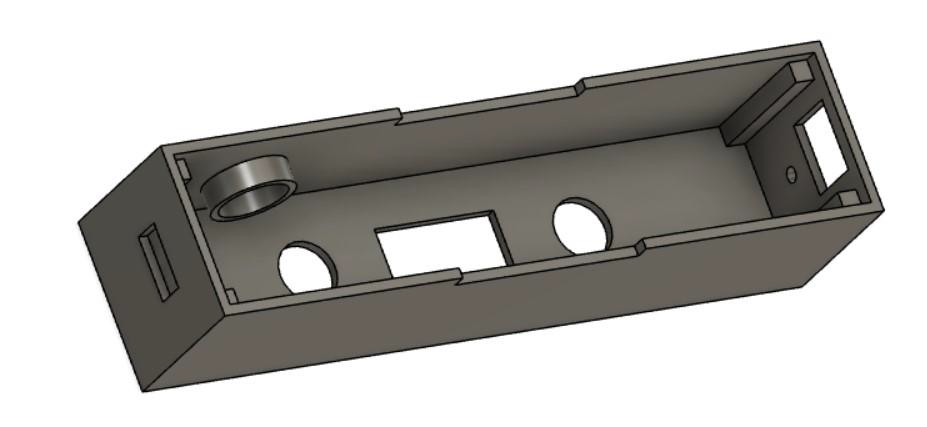(a) Inner Structure of the main 3D model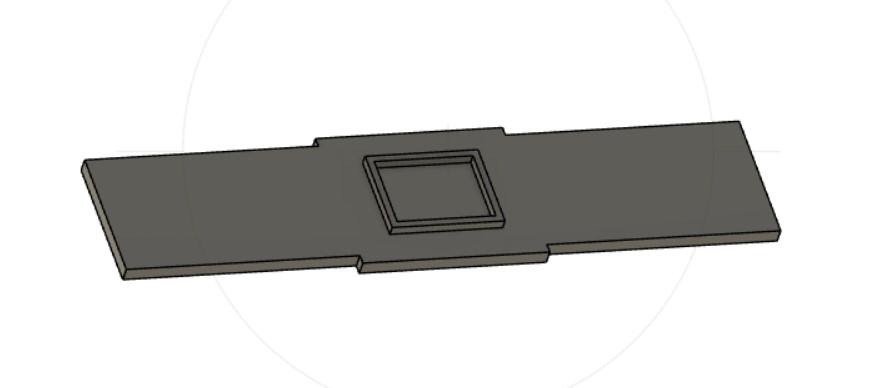(b) The lid of the main 3D model (removable)(c) Inside view of Final Built Spirit Level

ARDUINO CODE

```//Include Library
#include "Wire.h" //I2C Library
#include "I2Cdev.h"
#include "MPU6050.h" // MPU6050 Library
#include "math.h" // Math Library to use MATHS Functions
#include <Adafruit_SSD1306.h> //OLED library
#include <Fonts/FreeMonoBoldOblique12pt7b.h>
#include <Fonts/FreeSansBold12pt7b.h>
#include <Fonts/FreeMono12pt7b.h>
MPU6050 mpu; //Creating Object for MPU6050
#define SCREEN_WIDTH 128 // OLED display width, in pixels
#define SCREEN_HEIGHT 64 // OLED display height, in pixels
// Declaration for an SSD1306 display connected to I2C (SDA, SCL pins)
Adafruit_SSD1306 display(SCREEN_WIDTH, SCREEN_HEIGHT, &Wire, -1);
int push_button1 = 3;
int push_button2 = 4;
int buzzer = 5;
int battery_input = A0;
int16_t gyroX, gyroY, gyroZ, accX, accY, accZ, mpuTemp; // Variable to collect the raw data from MPU6050 Sensor
float CgyroX, CgyroY, CgyroZ, CaccX, CaccY, CaccZ; // Calibrated DATA variables
float Xang, Yang; // Acceleration Angle Variable
float FNXang, FOXang=0, FNYang, FOYang=0; //FILTER -->NEW-->OLD-->X or Y -->Acceleration ANGLE
float GXang=0, GYang=0; //Gyroscope Angle Variable
float ROLL, PITCH; //X-angle || Y-angle
float ROLL_REF=0,PITCH_REF=0;
float TEMP;
boolean set_gyro_angles = false, for_referance_data = false;
int Power;
int value1,value2;
int state=0,mode=0, ref_state=1;
int buzzer_value = 150;
float dt; // Delta T --> time gap between consecutive loops
unsigned long loop_timer; // Time Variable
void setup() {
Serial.begin(9600);
pinMode(A0,INPUT);
pinMode(push_button1,INPUT);
pinMode(push_button2,INPUT);
pinMode(buzzer,OUTPUT);
mpu.initialize(); //Initializing the MPU605
OFFSET();
if(!display.begin(SSD1306_SWITCHCAPVCC, 0x3C)) {
Serial.println(F("SSD1306 allocation failed"));
for(;;);
}
display.clearDisplay();
display.setTextColor(WHITE);
LOGO_DISPLAY();
loop_timer = micros() + 4000;
}
void loop() {
RAW_DATA();
ACC_ANGLE_CALCULATION();
GYRO_ANGLE_CALCULATION();
COMPLIMENTARY_FILTER();
// print data on push button toggling
while(value1 == 1 && state==0) {
analogWrite(buzzer,buzzer_value);
delay(20);
analogWrite(buzzer,0);
mode=1;
}
if(mode==1) {
display.invertDisplay(false);
display.setFont();
state=1;
ROLL_DISPLAY();
}
while(value1==1 && state==1 ) {
analogWrite(buzzer,buzzer_value);
delay(20);
analogWrite(buzzer,0);
mode=2;
}
if(mode==2) {
state=2;
PITCH_DISPLAY();
}
while(value1==1 && state==2 ) {
analogWrite(buzzer,buzzer_value);
delay(20);
analogWrite(buzzer,0);
mode=3;
}
if(mode==3) {
state = 3;
BOTH_ROLL_AND_PITCH_DISPLAY();
}
while(value1==1 && state==3 ) {
analogWrite(buzzer,buzzer_value);
delay(20);
analogWrite(buzzer,0);
mode=4;
}
if(mode == 4) {
state =4;
TEMP_DISPLAY();
}
while(value1==1 && state==4) {
analogWrite(buzzer,buzzer_value);
delay(20);
analogWrite(buzzer,0);
mode=1;
}
if(value2 == 1 && ref_state == 1) {
ROLL_REF = ROLL;
PITCH_REF = PITCH;
ref_state = 0;
while(value2 == 1) {
analogWrite(buzzer,buzzer_value);
delay(20);
analogWrite(buzzer,0);
}
}
if(value2 == 1 && ref_state == 0) {
ROLL_REF = 0;
PITCH_REF = 0;
ref_state = 1;
while(value2==1) {
analogWrite(buzzer,buzzer_value);
delay(20);
analogWrite(buzzer,0);
}
}
//set the frequency of void loop at 250Hz
while(loop_timer > micros());
loop_timer += 4000;
dt=0.004;
}
void OFFSET() {
mpu.setXAccelOffset(-1701);
mpu.setYAccelOffset(920);
mpu.setZAccelOffset(765);
mpu.setXGyroOffset(79);
mpu.setYGyroOffset(-20);
mpu.setZGyroOffset(-67);
}
void RAW_DATA() {
mpu.getMotion6(&accX, &accY, &accZ, &gyroX, &gyroY, &gyroZ);
}
//Function to calculate the angle from the RAW Accelerometer Date
void ACC_ANGLE_CALCULATION() {
//Angle Calculation --> Arc Tangent(X-gravity/Z-gravity)*(RADIAN TO DEGREE)
}
//Low pass filter to filter the noise coming from the disturbance
void LOW_PASS_FILTER() {
// [NEW_FILTER_DATA = a*OLD_FILTER_DATA + b*ACTUAL DATA] and [a + b = 1]
FNXang = (0.96*FOXang + 0.04*Xang); //ACCELEROMETER_ROLL
FNYang = (0.96*FOYang + 0.04*Yang); //ACCELEROMETER_PITCH
}
//To update the all previous data with new data
void UPDATE() {
//Updating the old filter value with new filter value
FOXang = FNXang;
FOYang = FNYang;
}
void GYRO_ANGLE_CALCULATION() {
// Converting the Raw Gyro Data in the range of +-250°/s
CgyroX = gyroX/131.0;
CgyroY = gyroY/131.0;
//Angle Calculation --> θ = θ + ω*Δt
GXang = GXang + CgyroX*dt - 0.0039;
GYang = GYang + CgyroY*dt + 0.00205;
}
void COMPLIMENTARY_FILTER() {
/* Gyro angle have drift but noise free and Acc angle have noise but no angle drift. Here we are making
complementary filters with GYRO AND ACC angles.
Gyro angle only works for a short period of time because Δt becomes zero if the sensor gets some stationary
position and works as a HIGH PASS FILTER.
While Acc angle will come out in long period or stationary system */
if(set_gyro_angles){ //If the IMU is already started
GXang = 0.94*GXang + 0.06*Xang;
GYang = 0.94*GYang + 0.06*Yang;
ROLL = GXang - ROLL_REF;
PITCH = GYang - PITCH_REF;
}
else { //At first start
GXang = Xang; //Set the gyro pitch angle equal to the accelerometer pitch
angle
GYang = Yang; //Set the gyro roll angle equal to the accelerometer roll
angle
set_gyro_angles = true; //Set the IMU started flag
}
}
void CALEBRATED_DATA() {
//To Get the GYRO DATA in the range of +-250°/s
CgyroX = gyroX/131.0;
CgyroY = gyroY/131.0;
CgyroZ = gyroZ/131.0;
//To get the ACC. DATA in the range of +-2g
CaccX = accX/16384.0;
CaccY = accY/16384.0;
CaccZ = accZ/16384.0;
}
void BOTH_ROLL_AND_PITCH_DISPLAY() {
display.clearDisplay();
// display temperature
display.setTextSize(1);
display.setCursor(0,0);
display.print("ROLL ");
display.setTextSize(2);
display.setCursor(0,10);
display.print(ROLL);
// display humidity
display.setTextSize(1);
display.setCursor(0, 35);
display.print("PITCH ");
display.setTextSize(2);
display.setCursor(0, 45);
display.print(PITCH);
BATTERY_LEVEL();
display.display();
}
void PITCH_DISPLAY() {
display.clearDisplay();
display.setFont(&FreeMono12pt7b);
display.setTextSize(1);
display.setCursor(0,25); //(35,0)
display.print("PITCH:");
display.setFont(&FreeSansBold12pt7b);
display.setTextSize(1);
display.setCursor(0, 55);
display.print(PITCH);
display.setFont();
BATTERY_LEVEL();
display.display();
}
void ROLL_DISPLAY() {
display.clearDisplay();
display.setFont(&FreeMono12pt7b);
display.setTextSize(1);
display.setCursor(0,25); //(35,0)
display.print("ROLL:");
display.setFont(&FreeSansBold12pt7b);
display.setTextSize(1);
display.setCursor(0, 55);
display.print(ROLL);
display.setFont();
BATTERY_LEVEL();
display.display();
}
void TEMP_DISPLAY() {
mpuTemp = mpu.getTemperature();
TEMP = mpuTemp/340.+36.53;
display.clearDisplay();
display.setFont(&FreeMono12pt7b);
display.setTextSize(1);
display.setCursor(0,25); //(35,0)
display.print("TEMP:");
display.setFont(&FreeSansBold12pt7b);
display.setTextSize(1);
display.setCursor(0, 55);
display.print(TEMP);
display.print(" C");
display.setFont();
BATTERY_LEVEL();
display.display();
}
void BATTERY_LEVEL() {
Power = map(analogRead(battery_input), 570,860,0,100);
display.setTextSize(1);
display.setCursor(100,0);
display.print(Power);
display.print("%");
//if(Power<20)
// {
// analogWrite(buzzer,buzzer_value);
// delay(500);
// analogWrite(buzzer,0);
// delay(200);
// }
}
void LOGO_DISPLAY() {
display.invertDisplay(true);
display.setFont(&FreeMonoBoldOblique12pt7b);
display.setTextSize(1);
display.setCursor(0,25);
display.print("Spirit");
display.setCursor(50,50);
display.print("Level");
display.display();
}
```
Diy projects